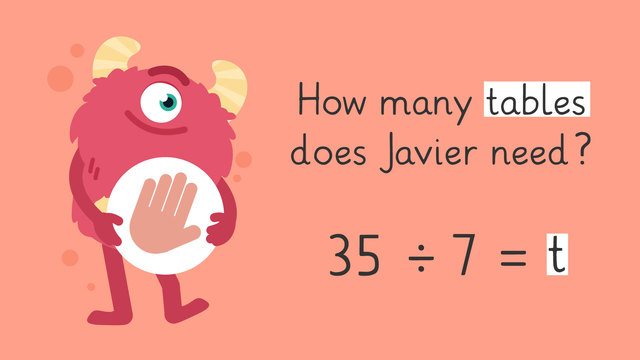# Solving Equations with Multiplication and Division—Let's Practice!Rating

Be the first to give a rating!
The authorsTeam Digital
Solving Equations with Multiplication and Division—Let's Practice!
CCSS.MATH.CONTENT.4.OA.A.2

## Basics on the topicSolving Equations with Multiplication and Division—Let's Practice!

Today we are solving equations using multiplication and division with Razzi! This video contains examples to help you further practice and grow confident in this topic.

### TranscriptSolving Equations with Multiplication and Division—Let's Practice!

Razzi says get these items ready (...) Because today we're going to practice... Solving Equations with Multiplication and Division. It's time to begin! Solve the word problem by writing an equation. Kiara's class is having a pizza party. There are eight pizzas and each pizza has six slices. How many slices are there in all? Pause the video to work on the problem (...) and press play when you are ready to see the solution! Eight times (...) six equals (...)

to represent the pizza. Then solve eight times six. Did you also get forty-eight slices of pizza? Let's tackle the next problem! Solve the word problem by writing an equation. Javier invited thirty-five friends to his birthday party and each table can fit seven seats. How many tables does Javier need? Pause the video to work on the problem (...) and press play when you are ready to see the solution! Thirty-five divided by (...) seven equals (...) to represent the tables. Then solve thirty-five divided by seven. Did you also get five tables? Let's try one more! Solve the word problem by writing an equation. Rosa has twelve points in her game. She played again and tripled her score. How many points does Rosa have now? Pause the video to work on the problem (...) and press play when you are ready to see the solution! Twelve times (...) three equals (...)

to represent the points. Then solve twelve times three. Did you also get thirty-six points? Razzi had so much fun practicing with you today! See you next time!

## Solving Equations with Multiplication and Division—Let's Practice! exercise

Would you like to apply the knowledge you’ve learned? You can review and practice it with the tasks for the video Solving Equations with Multiplication and Division—Let's Practice!.
• ### Determine if a problem is multiplication or division.

Hints

Splitting up a total equally is dividing. Adding the same amount multiple times is multiplication.

The word each is often a signal word for division word problems.

Making or producing something is often a signal word for multiplication word problems.

Solution
• The equation for word problem 1 should read 12 ÷ 3. This uses division.
• The equation for word problem 2 should read 40 ÷ 10. This uses division.
• The equation for word problem 3 should read 5 x 5. This uses multiplication.
• The equation for word problem 4 should read 5 x 4. This uses multiplication.
• ### Find the equation and solution.

Hints

Splitting up a total equally is dividing. Adding the same amount multiple times is multiplication.

The words double, triple, quadruple or times are often signal words for multiplication word problems.

Double means to multiply by 2 or add a number 2 times.

Solution
• Zuri scored 5 goals in the first game. In the second game she doubled her score.
• When you double something, it means to multiply by 2.
• The equation is 2 x 5 = 10. Zuri scored 10 goals her second game!
• ### Find the equation and solution.

Hints

Start by finding the total, then find the number you need to divide by.

There are 35 guests in total and you're dividing the guests into tables that seat 5.

The below image illustrates 35 people sitting 5 people to one table.

Solution

The image above illustrates the word problem.

Donna will need 7 tables. If there are 35 guest in total, and each table can sit 5 people, then the equation and solution is 35 ÷ 5 = 7.

• ### Matching word problems and equations.

Hints

Splitting up a total equally is dividing. Adding the same amount multiple times is multiplication.

The word each is often a signal word for division word problems.

The phrase all together is often a signal for multiplication word problems.

Solution
• 12 ÷ 2 = 6 housekeepers to clean each floor.
• 4 x 2 = 8 dollars for the wrapping paper.
• 16 ÷ 4 = 4 guest rooms for Eric's family.
• 4 x 3 = 12 firefighters in total.
• ### Find the equation and solution.

Hints

Splitting up a total equally is dividing. Adding the same amount multiple times is multiplication.

The words in all are often signal words for multiplication word problems.

Can you count the pieces of pizza to help you solve the problem?

Solution

The image above illustrates the word problem.

If there are 5 pizzas and each pizza has 6 slices, then the equation and solution is 5 x 6 = 30.

• ### Write and solve the equation.

Hints

Splitting up a total equally is dividing. Adding the same amount multiple times is multiplication.

The word each is often a signal word for division word problems.

Start with the total, then find the number you need to divide by.

There are 48 eggs in total and you're dividing the eggs into cartons that hold 6 eggs each.

The below image illustrates the word problem. Can you count how many containers there are?

Solution

The image above illustrates the word problem.

Malinda's family needs 8 egg containers. If there are 48 eggs in total, and each container can hold 6 eggs, then the equation and solution is 48 ÷ 6 = 8.